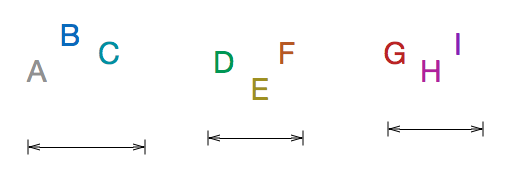# $\text{Min} \left ( \text{Max} \right )$

Logic Level 2The numbers from 1 to 9 (both inclusive) are divided into three groups with 3 numbers in each group . The numbers in each group are multiplied. The maximum of these numbers is chosen. Find the minimum value, this maximum can take.

×